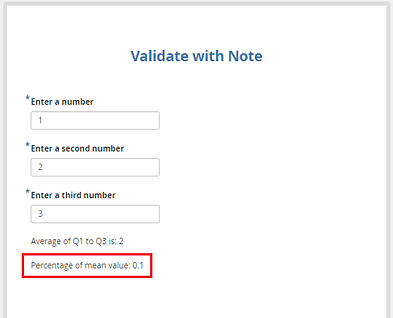# How to use the if-statement while building a survey form

Hi

@Kal_Lam Thanks a lot for your reponse.

Continue of this questions,
Q5: percentage of the mean value \2*5% = 0.1 (2 multiplied with 5% = 0.1) – working fine
Q6: Validation: \ we need to achieve a boundary condition.
For example:
Need to compare the value of Q1(enter a number) and Q4(Average of Q1 to Q3)
Q1: Enter a number = 1
Q4: Average of Q1 to Q3 = 2
so in between of 1 to 2( 1.1,1.2,1.3……….2.0) then Q6 should be populated with True if not ElseThanks!

Hi @keerthi,

Would you mind explaining me this a further so that we would be able to see if it’s possible.

Have a great day!

Hi,

Q1; Enter a number
Q2: Enter a second Number
Q3: Enter a third Number
Q4: Average of Q1 to Q3 is 2( as per the input of Q1 to Q3)
Q5:percentage of the mean value \2*5% = 0.1 (2 multiplied with 5% = 0.1)

Now need to achive the below format

Q6: Validation: \ it should display only message either true or false.

For example:
True or false condition is based on the Average of Q1 to Q3 and the Q1 (Enter a number).

If we enter 1 in Q1(Enter a number) and we have boundary conditions for exapmle(0 to 1.5).So the entered value is in between these boundary conditions is True or else False.Could you please responds ASAP. Thanks!

Hi @keerthi,

Do you mean you entered 1 in `Q1` (first number) and entered say 2 in `Q2` (last number). If the user enters say 1.1 or 1.3 or … which is in-between 1 to 2 for Q3 (number that comes in between Q1 to Q2) it should flag TRUE and if the user enters say 2.3 or a number like that it should flag FALSE?

Is this what you wish? If yes, would you also explain why you would need this is a survey (for my interest).

Have a great day!

Hi @Kal_Lam,

Yes, this is what we need.
As I’m beginger of this Kobotool box, so I’m trying in different format in creating forms by using Operations.

Thank you!

Hi @keerthi,

You could do the same by following the instructions outlined in the image below:

In the survey tab of your xlsform:

Data entry screen as seen in Enketo: for a condition not satisfying

Data entry screen as seen in Enketo: for a satisfying condition

Reference xlsform:
If Statement.xlsx (10.6 KB)

Have a great day!

1 Like